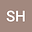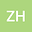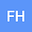Quasi-least square finite element methods for stationary incompressible magnetohydrodynamics problems
•••• +1
• Shahid Hussain,
• Zahid Hussain,
• Fazal Haq,
• Danping Yang
Shahid Hussain
Karakoram University

Corresponding Author:shahid8310@yahoo.com

Author ProfileZahid Hussain
Karakoram University
Author ProfileFazal Haq
Karakoram University
Author ProfileDanping Yang
East China Normal University
Author Profile## Abstract

This article aims to study the Quasi-least square mixed finite element (FE) method for the approximate solution of Magnato-Hydro-Dynamic equations (MHD). The resulting non-linear system of equations are linearized around a characteristic state, resulting in first order linearized least-square models that yield algebraic system of equations with symmetric positive definite coefficient matrices. A central feature of the method is that it does not require (Ladyzhenskaya-Babuska-Brezzi) LBB conditions on the finite dimensional subspaces and the resulting bilinear form is symmetric and positive definite. Secondly, it only needs to choose the value of a single parameter to find the well-posedness of the model equations. For the theoretical accuracy and authentication of the method, we investigate existence of the solutions and obtain a priori error estimates. The variables are fluid velocity, fluid pressure and magnetic field. Numerical tests are performed in order to assess the stability and the accuracy of the resulting methods. Result shows good agreement with analytical solutions.